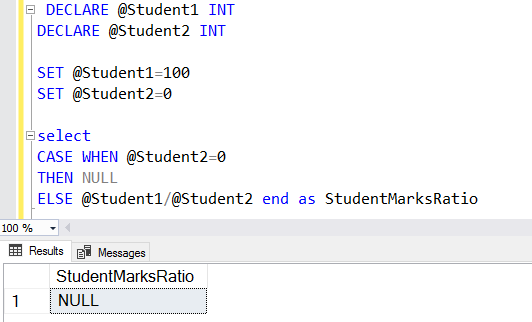A SQL CASE statement evaluates and returns results based on particular values, predicates and conditions as per defined logic. For example, suppose you have a voters table with the following details:

• Voter ID
• Name
• DOB

If you were looking for logic about voting eligibility, this would be dependent upon the values in the DOB column.

If a voter’s age is greater than 18, they are eligible to vote.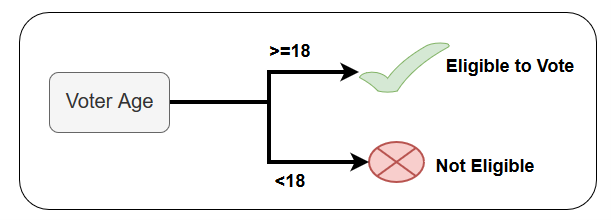Let’s look at another example. Many times, we store the column values in bits 1 or 0. Let’s say you store the values for a product’s availability as 1 or 0. For example:

• 1 = Product is available
• 0 = Product is out of stock

If we look at the database perspective, it is a good practice to use the abbreviations or bits wherever possible. It is beneficial for the SQL Server query optimizer in preparing the optimized execution plan. But, from the application perspective, the end-user does not require these values. Customers just need to view whether the product is available or not.

In the below image, we see both the database and application perspective.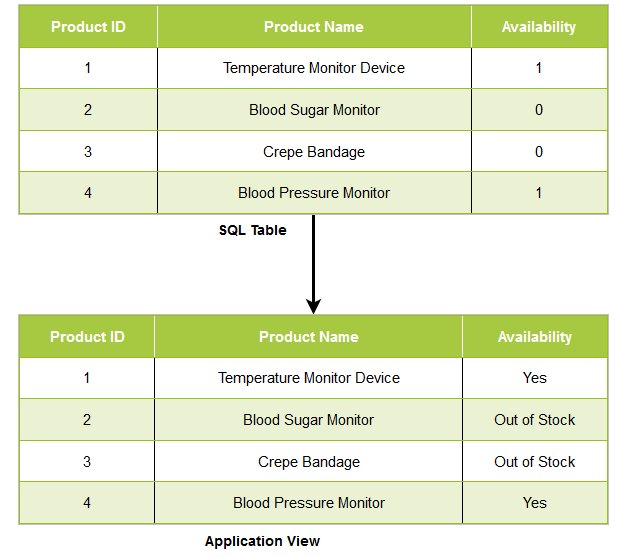## What does the SQL CASE statement do?

A CASE statement in SQL Server evaluates an expression and returns a value based on the defined conditions. Therefore, in the earlier example, the CASE statements work as shown below.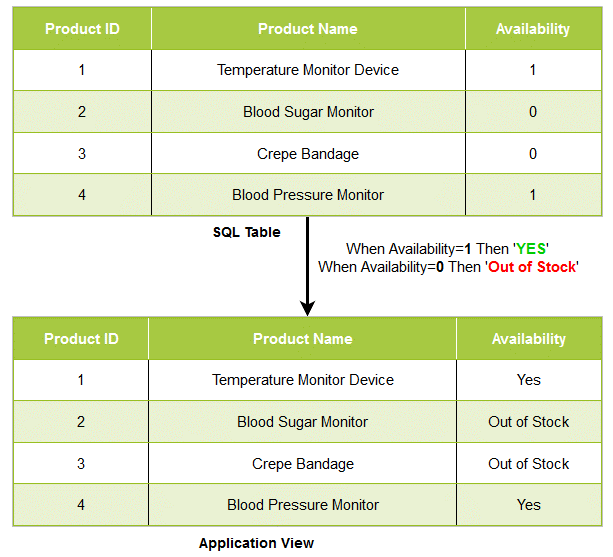At a high-level, the syntax for a SQL CASE statement is shown below. Here, we specified multiple conditions. SQL Server evaluates the conditions sequentially. Once a condition evaluates successfully, it stops the evaluation of remaining conditions. If none of the conditions are satisfied, we can use an optional ELSE statement to return the default value. For example, if we have a value different then 0 and 1 in the availability column, you get the output from the ELSE code block. It requires at least one set of the WHEN and THEN blocks. The CASE statement must end with the END block.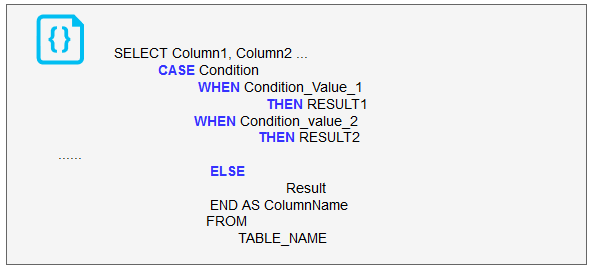Let’s explore the SQL CASE statement using various examples.

### The SELECT statement with a simple CASE expression

In this type of CASE statement, we use equality check expressions. The following query implements a simple CASE expression.

• If the value in the [SalariedFlag] is 1, then it shows the Active Employee
• For all other values, it displays the output as Inactive Employee
```SELECT TOP 5 Nationalidnumber ,
CASE salariedflag
WHEN 1 THEN 'Active Employee'
ELSE 'Inactive Employee'
END AS [Salaried Flag]We can specify multiple conditions for the CASE statement.

```SELECT TOP 5 Nationalidnumber ,
CASE salariedflag
WHEN 1 THEN 'Active Employee'
WHEN 0 THEN 'Inactive Employee'
ELSE 'Invalid Value'
END AS [Salaried Flag]

### Data standardization using SQL CASE statements

Usually, we use abbreviations to store values in SQL tables. The standard abbreviations are gender, country codes, marriage status, popular product names, etc.

Suppose we specify the abbreviations for storing employee genders. Now, our application should display the results without any abbreviations.

SQL CASE statements help to standardize the output for defined criteria. In the below query, we use the following conditions:

• If the gender value is M, display it as Male
• If the gender value is F, display it as Female
• For any other values, display Invalid Value
```SELECT DISTINCT CASE gender
WHEN 'M' THEN 'Male'
WHEN 'F' THEN 'Female'
ELSE 'Invalid Value'
END AS Gender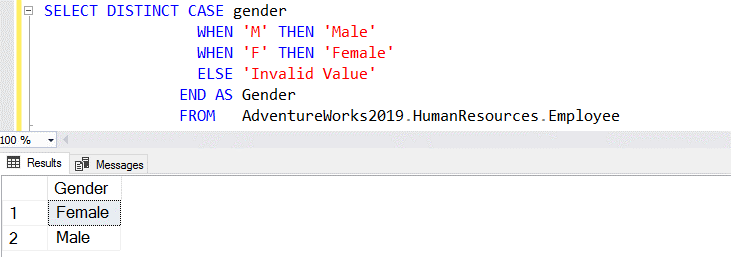### Searched CASE statements

In the searched CASE statement, we specify a CASE expression instead of the direct values. Once the expression value evaluates and satisfies a condition in the WHEN clause, its corresponding value is returned.

Look at the below SQL query. Here, we defined expressions in the WHEN clause for the [ListPrice]. It identifies that the product cost is \$250 and is marked as an Electronics item.

```SELECT ProductNumber, Name, [Product category] =
CASE
WHEN ListPrice = 0 THEN 'Out of Stock items'
WHEN ListPrice > 0 and ListPrice<=100 THEN 'Consumer goods'
WHEN ListPrice >100 and ListPrice <= 500 THEN 'Electronics items'
WHEN ListPrice >500 and ListPrice < 1500 THEN 'Luxury items'
ELSE 'Extra items'
END
FROM Production.Product order by ListPrice desc```

For the gender example referred to earlier, we can rewrite the SQL CASE statement for the gender abbreviations using the searched case statements.

```SELECT DISTINCT CASE
WHEN Gender='M' THEN 'Male'
WHEN Gender='F' THEN 'Female'
ELSE 'Invalid Value'
END AS Gender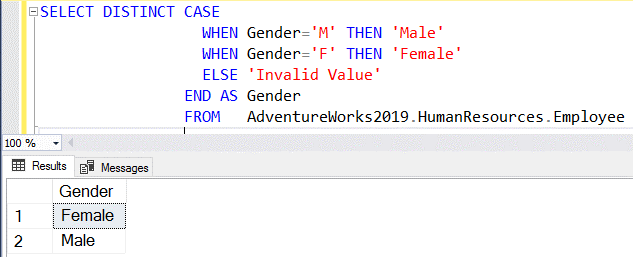### Using CASE statements with the ORDER BY clause

SQL queries use the ORDER BY clause for data sorting in either ascending or descending order. You can use the CASE statements in conjunction with the ORDER BY clause. Suppose from the products table, we retrieve the [ProductName] and [ListPrice]. We want to sort the results in the following ways:

• If the product list price is less than 2,000, you want the result in the default sort order, i.e., ascending
• If the product list price is greater than 2,000, the ORDER BY clause sort results in descending order

In this query, we use two SQL CASE statements to implement the logic.

```SELECT Name,
ListPrice
FROM Production.Product
ORDER BY CASE
WHEN ListPrice<=2000 THEN ListPrice END
,CASE WHEN ListPrice >2000 THEN ListPrice END DESC```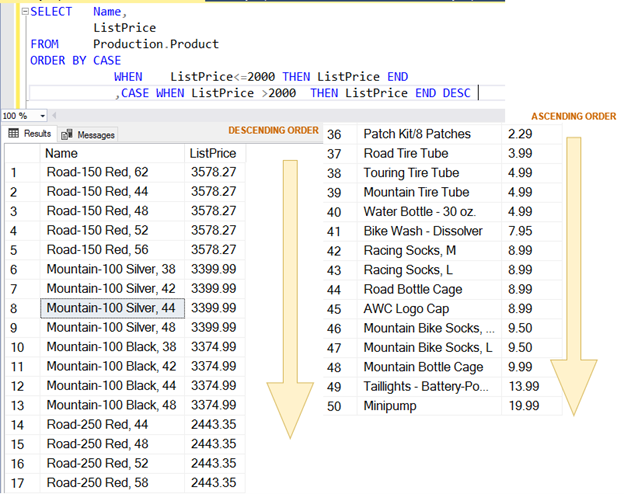In the below query output, you can verify the data sorts appearing in both descending and ascending order.

In another example, suppose we want to sort data in the employee table based on the following condition:

• For active employees (Current flag =1), data should sort the hire date column
• For inactive employees, it should sort data as per the values in the birthdate column
```SELECT NationalIDNumber,JobTitle,Hiredate,BirthDate, currentflag
ORDER BY
CASE CURRENTFLAG WHEN 1 THEN HireDate
else Birthdate
end```

In the query output, we can verify the data sort order defined by the ORDER BY clause and CASE statements.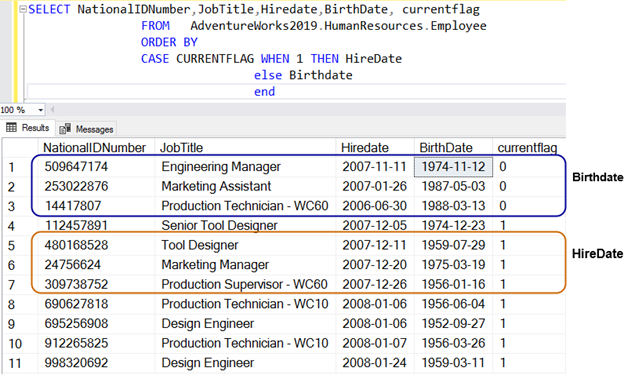### CASE statement in SQL and aggregate functions

Aggregate functions in SQL Server perform calculations and return a single value. Examples of aggregate functions are MIN, MAX, COUNT, ABG and CHECKSUM.

Suppose we want to retrieve the employee hire count for each year from 2007-2010. It should display results in the following format: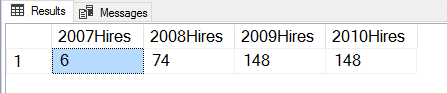For this purpose, we use the COUNT aggregate function in SQL Server.

• First, the SQL DATEPART function filters data according to the year. For example, the DATEPART(YY, Hiredate)=2007, filters data for the year 2007.
• We then use the CASE statement to return 1 if the year is 2007.
• The count aggregate function counts the records and displays the results.
• Similarly, the query works for the remaining years.
```SELECT Count(CASE
WHEN Datepart(yy, hiredate) = 2007 THEN 1
ELSE NULL
END) AS [2007Hires],
Count(CASE
WHEN Datepart(yy, hiredate) = 2008 THEN 1
ELSE NULL
END) AS [2008Hires],
Count(CASE
WHEN Datepart(yy, hiredate) = 2009 THEN 1
ELSE NULL
END) AS [2009Hires],
Count(CASE
WHEN Datepart(yy, hiredate) = 2009 THEN 1
ELSE NULL
END) AS [2010Hires]

Similarly, let’s say we want to use the aggregate function GROUP BY to group rows having the same product category. We can specify the CASE statement in SQL to sort data from the grouped result set.

```SELECT [Product category] = CASE
WHEN listprice = 0 THEN 'Out of Stock items'
WHEN listprice > 0
AND listprice <= 100 THEN 'Consumer goods'
WHEN listprice > 100
AND listprice <= 500 THEN 'Electronics items'
WHEN listprice > 500
AND listprice < 1500 THEN 'Luxury items'
ELSE 'Extra items'
END,
Min(listprice) AS MinPrice,
Max(listprice) AS MaxPrice,
Count(listprice) AS Numberofproducts
FROM production.product
GROUP BY CASE
WHEN listprice = 0 THEN 'Out of Stock items'
WHEN listprice > 0
AND listprice <= 100 THEN 'Consumer goods'
WHEN listprice > 100
AND listprice <= 500 THEN 'Electronics items'
WHEN listprice > 500
AND listprice < 1500 THEN 'Luxury items'
ELSE 'Extra items'
END
ORDER BY numberofproducts DESC```

In the above query, we use two SQL CASE statements.

• The first CASE statement categorizes the data based on the expression defined in the list price. Using this CASE statement, we divide the products into the following categories:
• Out of Stock items
• Consumer goods
• Electronic items
• Luxury items
• In the second case statement, we use the GROUP BY aggregate function to group the result by the category
• Further, we sort the results as per the NumberOfProducts in the descending order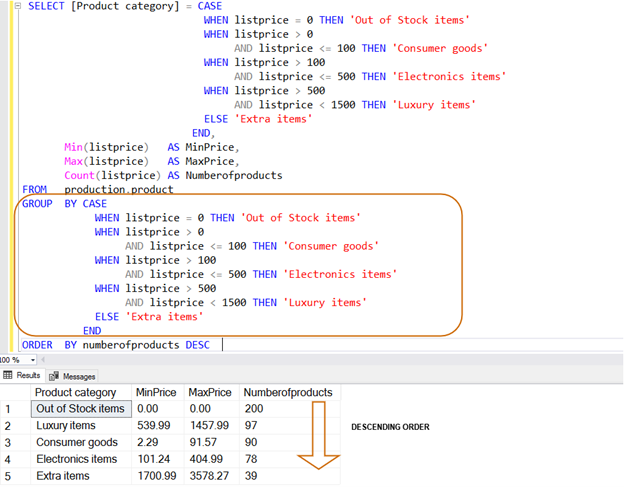### Prevent divide by zero error using SQL CASE statements

A divide by zero error occurs if the denominator value is zero. If you do these fractions in SQL Server, it will give you the divide by zero error as shown below.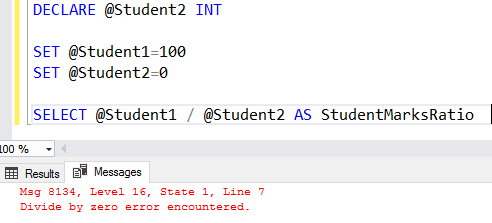It is an excellent practice to write your queries in a way to avoid these common mistakes. To avoid this, we use the fraction logic inside a CASE statement.

```DECLARE @Student1 INT
DECLARE @Student2 INT

SET @Student1=100
SET @Student2=0

select
CASE WHEN @Student2=0
THEN NULL
ELSE @Student1/@Student2 end as StudentMarksRatio```

We have safeguarded our query from the divide by zero error. Now, with the modified logic, if we get a zero in the denominator, you get NULL in the output as shown below.